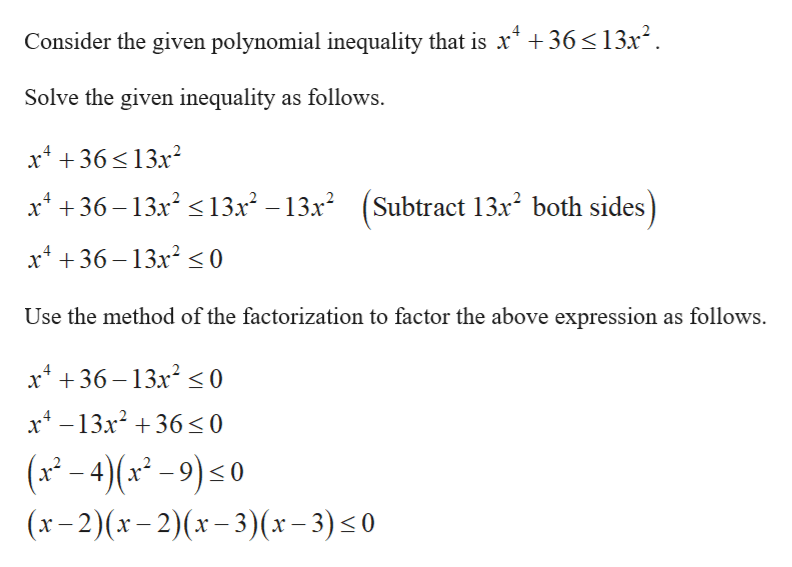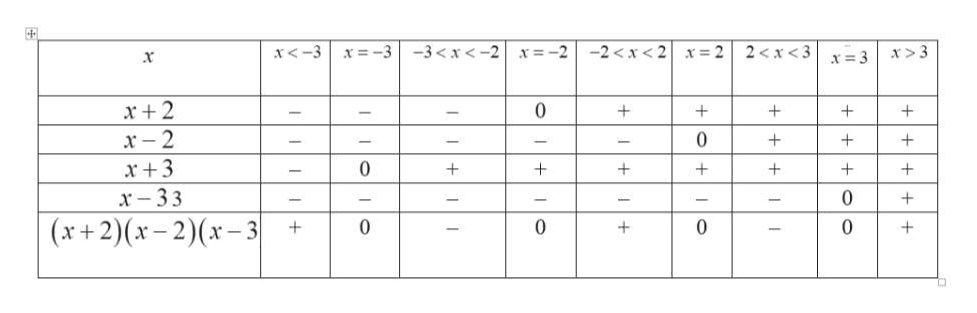# How do you solve the polynomial inequality x4 + 36 ≤ 13x2

Question
11 views

How do you solve the polynomial inequality x+ 36 ≤ 13x2

check_circle

Step 1

The given polynomial inequality is x^4+36≤-13x^2.

Step 2help_outlineImage Transcriptionclose4 Consider the given polynomial inequality that is x 36 13x Solve the given inequality as follows x4 36132 (Subtract 13x2 both sides) x36-132 132 - 13x2 x4 36 13x2 0 Use the method of the factorization to factor the above expression as follows x36 13x2< 0 x4-13x2 36 0 (x-4(x9)o (x-2)x-2) (x-3)(x-3) 0 0 fullscreen
Step 3

Construct a sign chart table to identify the intervals that s...help_outlineImage Transcriptionclose-23 x=3 x +2 + x -2 x +3 + x -33 0 + - (x+2)(x-2) (x-3| fullscreen

### Want to see the full answer?

See Solution

#### Want to see this answer and more?

Solutions are written by subject experts who are available 24/7. Questions are typically answered within 1 hour.*

See Solution
*Response times may vary by subject and question.
Tagged in

### Algebra